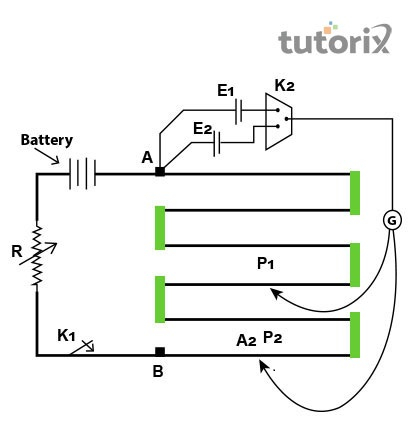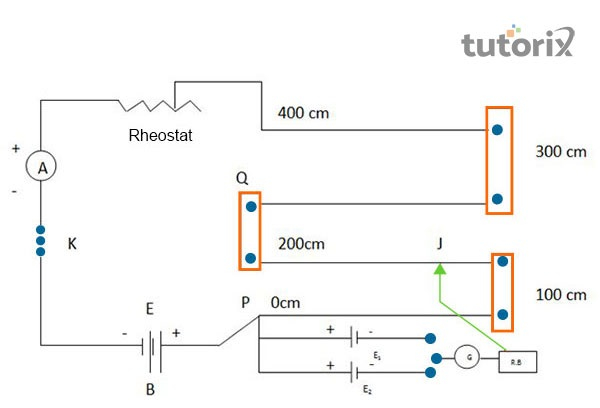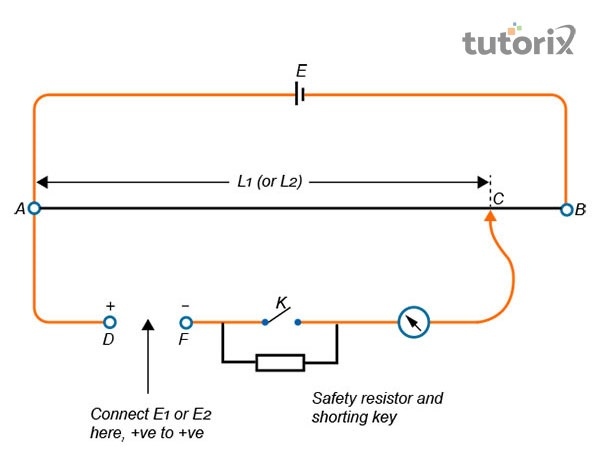# To compare the emf of two given primary cells using potentiometer experiment

## Introduction

Potentiometer experiment appears as a regular test for comparing the electromotive force (EMF) of two given primary cells.

This experiment is commonly used for saving the external environment with its measurement and comparison of the EMF of two primary cells at a given time. The primary cell is categorised between the Daniel cells and the Leclanche cells which allows the assessment of the EMF of two cells in real-time.

## What is Potentiometer Experiment?

Potentiometer experiment is conducted through a device that is used for measuring the potential differences within a circuit.

The potentiometer is mostly used for measuring the potential difference that brings charge from one specific point to another (Palomino-Ruiz et al. 2021).

In general, two types of potentiometer can be traced, the faders potentiometer and the rotary potentiometer for comparing the EMF within two given primary cells.Figure 1: Potentiometer Experiment

The faders potentiometers are mostly used in the modern low-powered audio systems. These potentiometers serves as the audio control device for controlling the attenuation of frequencies within the devices (Thefactfactor, 2022).

The rotary potentiometer on the other hand is commonly used for controlling the loudness and shaping the characteristics of audio signals in real-time. In simple words, the potentiometer appears as a crucial device that can help in measuring and comparing.

### Materials required for the Potentiometer Experiment

The potentiometer experiment is an effective test used for comparing the EMF of two primary cells within a certain circuit. The experiment follows a series of materials or apparatus in order to identify the EMF differences between two primary cells within a certain circuit.

• The first and possibly the most essential component of this experiment in this test is a potentiometer that will be used for measuring the EMF of two cells (Shah et al. 2019).

• Then two cells are needed to conduct the test in real-time. The two cells used in this experiment are the Daniel cell and the Leclanche cell.

• Then a low resistance Rheostat is used to stabilize the experiment within the circuits and the cells where current flows.

• After that, three crucial apparatus, an ammeter, a voltmeter and a galvanometer will be used in order to form the one-way key’ and the two-way key in real-time.

• Then some commonly available materials like jockey, set square and resistance box are required to conduct the test in real-time.

• Finally, some connecting wires and a piece of sandpaper are needed to successfully conduct the test while comparing the EMF of two primary cells.

## Theory for comparing the EMF of two cells using Potentiometer Experiment

In a potentiometer experiment the potential differences between the terminal cells present within the circuits can be measured by using a voltmeter in the experiment.

The potentiometer on the other hand, is used for determining the value of EMF of two primary cells that are present within the test (Physicsmax, 2022).

Let us assume that $\mathrm{E_1}$ and $\mathrm{E_2}$ are the two primary cells here and the EMF of these two cells is $\mathrm{I_1}$ and $\mathrm{I_2}$.Figure 2: Circuit diagram for Potentiometer Experiment

The given EMF is directed to balance the lengths of the $\mathrm{E_1}$ and $\mathrm{E_2}$ cells when the diagram is respectively connected to the circuit. In this experiment,$\mathrm{\varphi}$ represents the potential gradient that comes along with the potentiometer wire in the real-time experiment. The formula used in this theory will be $\mathrm{E_1/E_2= \varphi\: I_1/\: \varphi \:I_2 = I_1/I_2}$.

## Procedures involved in the Potentiometer Experiment

The procedure for the potentiometer experiment follows an extensive pathway where different materials and different processes are involved at the same time. First, the apparatus required for the experiment needs to be arranged properly in the circuit diagram. After that, the positive poles needs to be connected to the negative poles and the terminal poles.Figure 3: Compare EMFs in two cells using the potentiometer

After that, the plug between the two-way key needs to be inserted and the jockey needs to be slid gently over the wires of the potentiometer (Physicsmax, 2022). Finally, the notes need to be made based on the length of the point and everything visible in the null point needs to be recorded for observing the resistance difference in the experiment.

## Observations of the Potentiometer Experiment

Balancing Lengths$\mathrm{E_1/E_2 = l_A J_1/ l_A j_2}$
$\mathrm{L_1}$ for cell $\mathrm{E_1}$ (cm)$\mathrm{L_2}$ for cell $\mathrm{E_2}$ (cm)
3273760.86
323.53710.87
321.53690.87
312.5352.50.88

Table 1: Observation Table

In the above table, the final ratio of the EMF is $\mathrm{E_1/E_2\:\sim\:0.87}$ which is also the result of the above observation.

## Conclusion

The potentiometer experiment appears as an effective test that can compare the EMF of two cells properly. When current flows through circuits, the potentiometer is used for measuring the internal resistance of the current flowing within the circuit. With all its required apparatus, the potentiometer experiment is capable of measuring the EMF difference between primary cells successfully.

Q1. What are some crucial precautions used in the potentiometer experiment?

Ans: While doing the potentiometer experiment the connection needs to be tight, neat and clean. In addition, all the positive polls of the used battery in this experiment need to be connected to the terminal of the wires.

Q2. What are the possible sources of errors present in the potentiometer experiment?

Ans: In a potentiometer experiment, the battery may not be charged properly, and the potentiometer wire may not be made with high-class materials and uniform cross-section. There are also chances that the end resistance may not be at 0 throughout the length of the wire.

Q3. Which principle actively works behind the potentiometer experiment?

Ans: The principle of operation is an active part of the potentiometer experiment as it relies on the uniform cross-section that carries a constant current within the process. The principle states that the length of the current and its constant flow are directly proportional to each other.